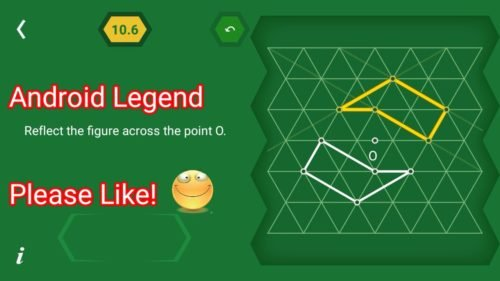# Pythagorea 60 degree Level 10.1 10.2 10.3 10.4 10.5 10.6 10.7 10.8 10.9 10.10 10.11 Solution/AnswersSolutions, Hints, and Answers to pythagorea 60° are available in this post, Scroll down to find solutions to all the levels.

Pythagorea sixty degrees is android/iOS app developed by Horis International Limited. This game is mostly focused on geometric puzzles and construction. The workspace is divided into triangular grids lines aligned 60° to each other, that’s why this game names as pythagorea 60°. You should know all the basic Math operations. All lines and shapes are drawn on a grid whose cells are equilateral triangles. Most of the game levels can be answered using natural intuition and bye some basic laws of geometry.

You have to connect points on the grid using straight lines to construct an element, you can even use intersection points to draw. some levels are very easy some are of medium difficulty and some are very hard to solve, that’s why I am providing solutions to all the problems.

If you are here for levels other than ‘Point Symmetry’ Go to directory of all other levels at : http://www.puzzlegamemaster.com/pythagorea-60-complete/

• Pythagorea 60° Level 10.1: Reflect the polyline across the point O.• Pythagorea 60° Level 10.2: Reflect the triangle across the point O.• Pythagorea 60° Level 10.3: Construct the center of symmetry of the given figure.• Pythagorea 60° Level 10.4: Select two points that are symmetric With respect to the point O.• Pythagorea 60° Level 10.5: Construct the center of symmetry of the given figure.• Pythagorea 60° Level 10.6: Reflect the figure across the point O.• Pythagorea 60° Level 10.7: Reflect the polyline across the point O.• Pythagorea 60° Level 10.8: Reflect the polyline across the point O.• Pythagorea 60° Level 10.9: Reflect the figure across the midpoint of the Side AB.• Pythagorea 60° Level 10.10: Reflect the figure across the midpoint of the Side AB.• Pythagorea 60° Level 10.11: Construct the center of symmetry of the given figure.Feel free to comment below if you have any doubts regarding the solutions. I will try to help you guys. All other levels are posted on this blog please visit them too.